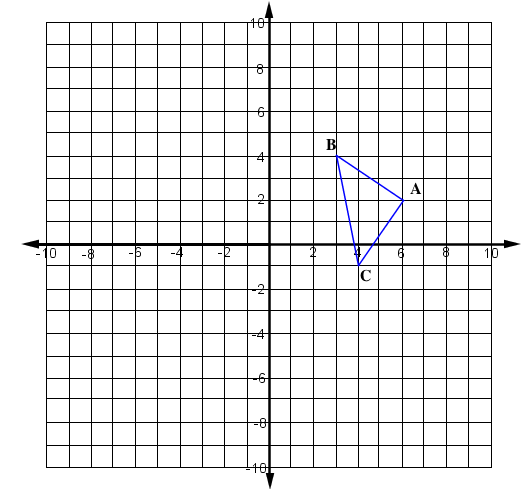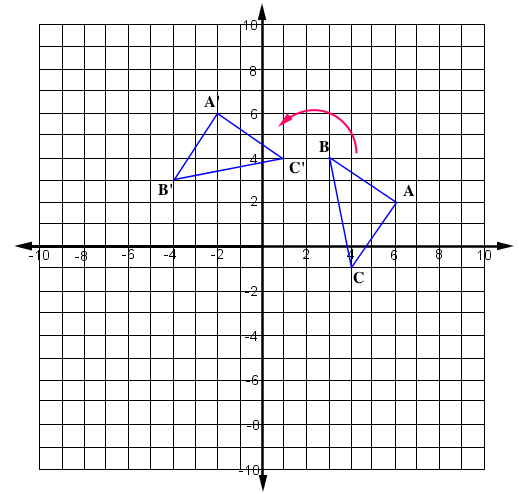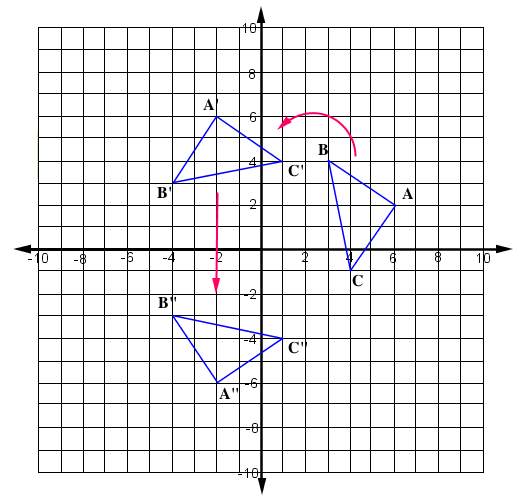### Home > GC > Chapter 12 > Lesson 12.2.3 > Problem12-81

12-81.

Multiple Choice: Assume that $A\left(6,2\right)$, $B\left(3,4\right)$, and $C\left(4,-1\right)$. If $\Delta ABC$ is rotated $90º$ counterclockwise ($\circlearrowleft$) to form $\Delta A'B'C'$, and if $\Delta A'B'C'$ is reflected across the $x$-axis to form $\Delta A''B''C''$, then the coordinates of $C''$ are:

1. $\left(1,4\right)$

1. $\left(-4,1\right)$

1. $\left(1,-4\right)$

1. $\left(4,1\right)$

Graph $\Delta ABC$.

Rotate the triangle $90^\circ$ counterclockwise.

Reflect the triangle across the $x$-axis and then find the coordinates of the new triangle.Click the link at right for the full version of the eTool: 12-81 HW eTool (Desmos)
Use this eTool to find the coordinate of C".